# JP5562870B2 - Hybrid work machine and control method thereof - Google Patents

## Info

Publication number
JP5562870B2
JP5562870B2 JP2010546661A JP2010546661A JP5562870B2 JP 5562870 B2 JP5562870 B2 JP 5562870B2 JP 2010546661 A JP2010546661 A JP 2010546661A JP 2010546661 A JP2010546661 A JP 2010546661A JP 5562870 B2 JP5562870 B2 JP 5562870B2
Authority
JP
Japan
Prior art keywords
output
hydraulic pump
hydraulic
pump
pressure
Prior art date
Legal status (The legal status is an assumption and is not a legal conclusion. Google has not performed a legal analysis and makes no representation as to the accuracy of the status listed.)
Active
Application number
JP2010546661A
Other languages
Japanese (ja)
Other versions
JPWO2010082636A1 (en
Inventor

Original Assignee

Priority date (The priority date is an assumption and is not a legal conclusion. Google has not performed a legal analysis and makes no representation as to the accuracy of the date listed.)
Filing date
Publication date
Priority to JP2009007949 priority Critical
Priority to JP2009007949 priority
Priority to JP2009142638 priority
Priority to JP2009142638 priority
Application filed by 住友重機械工業株式会社 filed Critical 住友重機械工業株式会社
Priority to JP2010546661A priority patent/JP5562870B2/en
Priority to PCT/JP2010/050434 priority patent/WO2010082636A1/en
Publication of JPWO2010082636A1 publication Critical patent/JPWO2010082636A1/en
Application granted granted Critical
Publication of JP5562870B2 publication Critical patent/JP5562870B2/en
Application status is Active legal-status Critical
Anticipated expiration legal-status Critical

## Images

•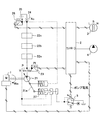•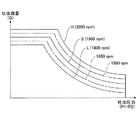••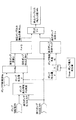•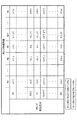•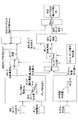•••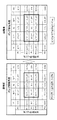••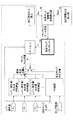•••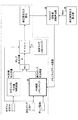••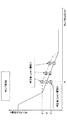•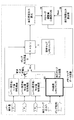•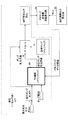•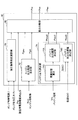•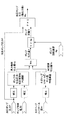•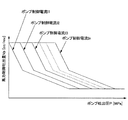•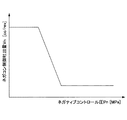•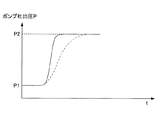•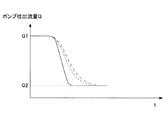•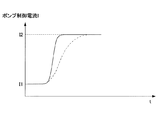•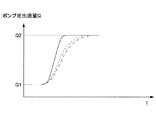••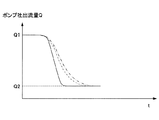•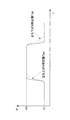•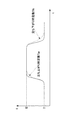•## Classifications

• BPERFORMING OPERATIONS; TRANSPORTING
• B60VEHICLES IN GENERAL
• B60WCONJOINT CONTROL OF VEHICLE SUB-UNITS OF DIFFERENT TYPE OR DIFFERENT FUNCTION; CONTROL SYSTEMS SPECIALLY ADAPTED FOR HYBRID VEHICLES; ROAD VEHICLE DRIVE CONTROL SYSTEMS FOR PURPOSES NOT RELATED TO THE CONTROL OF A PARTICULAR SUB-UNIT
• B60W10/00Conjoint control of vehicle sub-units of different type or different function
• B60W10/30Conjoint control of vehicle sub-units of different type or different function including control of auxiliary equipment, e.g. air-conditioning compressors or oil pumps
• BPERFORMING OPERATIONS; TRANSPORTING
• B60VEHICLES IN GENERAL
• B60LPROPULSION OF ELECTRICALLY-PROPELLED VEHICLES; SUPPLYING ELECTRIC POWER FOR AUXILIARY EQUIPMENT OF ELECTRICALLY-PROPELLED VEHICLES; ELECTRODYNAMIC BRAKE SYSTEMS FOR VEHICLES IN GENERAL; MAGNETIC SUSPENSION OR LEVITATION FOR VEHICLES; MONITORING OPERATING VARIABLES OF ELECTRICALLY-PROPELLED VEHICLES; ELECTRIC SAFETY DEVICES FOR ELECTRICALLY-PROPELLED VEHICLES
• B60L1/00Supplying electric power to auxiliary equipment of vehicles
• B60L1/003Supplying electric power to auxiliary equipment of vehicles to auxiliary motors, e.g. for pumps, compressors
• BPERFORMING OPERATIONS; TRANSPORTING
• B60VEHICLES IN GENERAL
• B60LPROPULSION OF ELECTRICALLY-PROPELLED VEHICLES; SUPPLYING ELECTRIC POWER FOR AUXILIARY EQUIPMENT OF ELECTRICALLY-PROPELLED VEHICLES; ELECTRODYNAMIC BRAKE SYSTEMS FOR VEHICLES IN GENERAL; MAGNETIC SUSPENSION OR LEVITATION FOR VEHICLES; MONITORING OPERATING VARIABLES OF ELECTRICALLY-PROPELLED VEHICLES; ELECTRIC SAFETY DEVICES FOR ELECTRICALLY-PROPELLED VEHICLES
• B60L50/00Electric propulsion with power supplied within the vehicle
• B60L50/10Electric propulsion with power supplied within the vehicle using propulsion power supplied by engine-driven generators, e.g. generators driven by combustion engines
• B60L50/16Electric propulsion with power supplied within the vehicle using propulsion power supplied by engine-driven generators, e.g. generators driven by combustion engines with provision for separate direct mechanical propulsion
• BPERFORMING OPERATIONS; TRANSPORTING
• B60VEHICLES IN GENERAL
• B60WCONJOINT CONTROL OF VEHICLE SUB-UNITS OF DIFFERENT TYPE OR DIFFERENT FUNCTION; CONTROL SYSTEMS SPECIALLY ADAPTED FOR HYBRID VEHICLES; ROAD VEHICLE DRIVE CONTROL SYSTEMS FOR PURPOSES NOT RELATED TO THE CONTROL OF A PARTICULAR SUB-UNIT
• B60W10/00Conjoint control of vehicle sub-units of different type or different function
• B60W10/04Conjoint control of vehicle sub-units of different type or different function including control of propulsion units
• B60W10/06Conjoint control of vehicle sub-units of different type or different function including control of propulsion units including control of combustion engines
• BPERFORMING OPERATIONS; TRANSPORTING
• B60VEHICLES IN GENERAL
• B60WCONJOINT CONTROL OF VEHICLE SUB-UNITS OF DIFFERENT TYPE OR DIFFERENT FUNCTION; CONTROL SYSTEMS SPECIALLY ADAPTED FOR HYBRID VEHICLES; ROAD VEHICLE DRIVE CONTROL SYSTEMS FOR PURPOSES NOT RELATED TO THE CONTROL OF A PARTICULAR SUB-UNIT
• B60W10/00Conjoint control of vehicle sub-units of different type or different function
• B60W10/04Conjoint control of vehicle sub-units of different type or different function including control of propulsion units
• B60W10/08Conjoint control of vehicle sub-units of different type or different function including control of propulsion units including control of electric propulsion units, e.g. motors or generators
• BPERFORMING OPERATIONS; TRANSPORTING
• B60VEHICLES IN GENERAL
• B60WCONJOINT CONTROL OF VEHICLE SUB-UNITS OF DIFFERENT TYPE OR DIFFERENT FUNCTION; CONTROL SYSTEMS SPECIALLY ADAPTED FOR HYBRID VEHICLES; ROAD VEHICLE DRIVE CONTROL SYSTEMS FOR PURPOSES NOT RELATED TO THE CONTROL OF A PARTICULAR SUB-UNIT
• B60W20/00Control systems specially adapted for hybrid vehicles
• EFIXED CONSTRUCTIONS
• E02HYDRAULIC ENGINEERING; FOUNDATIONS; SOIL SHIFTING
• E02FDREDGING; SOIL-SHIFTING
• E02F9/00Component parts of dredgers or soil-shifting machines, not restricted to one of the kinds covered by groups E02F3/00 - E02F7/00
• E02F9/20Drives; Control devices
• E02F9/2058Electric or electro-mechanical or mechanical control devices of vehicle sub-units
• E02F9/2062Control of propulsion units
• E02F9/2075Control of propulsion units of the hybrid type
• EFIXED CONSTRUCTIONS
• E02HYDRAULIC ENGINEERING; FOUNDATIONS; SOIL SHIFTING
• E02FDREDGING; SOIL-SHIFTING
• E02F9/00Component parts of dredgers or soil-shifting machines, not restricted to one of the kinds covered by groups E02F3/00 - E02F7/00
• E02F9/20Drives; Control devices
• E02F9/22Hydraulic or pneumatic drives
• E02F9/2221Control of flow rate; Load sensing arrangements
• E02F9/2232Control of flow rate; Load sensing arrangements using one or more variable displacement pumps
• E02F9/2235Control of flow rate; Load sensing arrangements using one or more variable displacement pumps including an electronic controller
• EFIXED CONSTRUCTIONS
• E02HYDRAULIC ENGINEERING; FOUNDATIONS; SOIL SHIFTING
• E02FDREDGING; SOIL-SHIFTING
• E02F9/00Component parts of dredgers or soil-shifting machines, not restricted to one of the kinds covered by groups E02F3/00 - E02F7/00
• E02F9/20Drives; Control devices
• E02F9/22Hydraulic or pneumatic drives
• E02F9/2246Control of prime movers, e.g. depending on the hydraulic load of work tools
• BPERFORMING OPERATIONS; TRANSPORTING
• B60VEHICLES IN GENERAL
• B60WCONJOINT CONTROL OF VEHICLE SUB-UNITS OF DIFFERENT TYPE OR DIFFERENT FUNCTION; CONTROL SYSTEMS SPECIALLY ADAPTED FOR HYBRID VEHICLES; ROAD VEHICLE DRIVE CONTROL SYSTEMS FOR PURPOSES NOT RELATED TO THE CONTROL OF A PARTICULAR SUB-UNIT
• B60W2300/00Indexing codes relating to the type of vehicle
• B60W2300/17Construction vehicles, e.g. graders, excavators
• BPERFORMING OPERATIONS; TRANSPORTING
• B60VEHICLES IN GENERAL
• B60WCONJOINT CONTROL OF VEHICLE SUB-UNITS OF DIFFERENT TYPE OR DIFFERENT FUNCTION; CONTROL SYSTEMS SPECIALLY ADAPTED FOR HYBRID VEHICLES; ROAD VEHICLE DRIVE CONTROL SYSTEMS FOR PURPOSES NOT RELATED TO THE CONTROL OF A PARTICULAR SUB-UNIT
• B60W2510/00Input parameters relating to a particular sub-units
• B60W2510/30Auxiliary equipments
• B60W2510/305Power absorbed by auxiliaries
• YGENERAL TAGGING OF NEW TECHNOLOGICAL DEVELOPMENTS; GENERAL TAGGING OF CROSS-SECTIONAL TECHNOLOGIES SPANNING OVER SEVERAL SECTIONS OF THE IPC; TECHNICAL SUBJECTS COVERED BY FORMER USPC CROSS-REFERENCE ART COLLECTIONS [XRACs] AND DIGESTS
• Y02TECHNOLOGIES OR APPLICATIONS FOR MITIGATION OR ADAPTATION AGAINST CLIMATE CHANGE
• Y02TCLIMATE CHANGE MITIGATION TECHNOLOGIES RELATED TO TRANSPORTATION
• Y02T10/00Road transport of goods or passengers
• Y02T10/60Other road transportation technologies with climate change mitigation effect
• Y02T10/62Hybrid vehicles
• Y02T10/6286Control systems for power distribution between ICE and other motor or motors
• YGENERAL TAGGING OF NEW TECHNOLOGICAL DEVELOPMENTS; GENERAL TAGGING OF CROSS-SECTIONAL TECHNOLOGIES SPANNING OVER SEVERAL SECTIONS OF THE IPC; TECHNICAL SUBJECTS COVERED BY FORMER USPC CROSS-REFERENCE ART COLLECTIONS [XRACs] AND DIGESTS
• Y02TECHNOLOGIES OR APPLICATIONS FOR MITIGATION OR ADAPTATION AGAINST CLIMATE CHANGE
• Y02TCLIMATE CHANGE MITIGATION TECHNOLOGIES RELATED TO TRANSPORTATION
• Y02T10/00Road transport of goods or passengers
• Y02T10/60Other road transportation technologies with climate change mitigation effect
• Y02T10/70Energy storage for electromobility
• YGENERAL TAGGING OF NEW TECHNOLOGICAL DEVELOPMENTS; GENERAL TAGGING OF CROSS-SECTIONAL TECHNOLOGIES SPANNING OVER SEVERAL SECTIONS OF THE IPC; TECHNICAL SUBJECTS COVERED BY FORMER USPC CROSS-REFERENCE ART COLLECTIONS [XRACs] AND DIGESTS
• Y02TECHNOLOGIES OR APPLICATIONS FOR MITIGATION OR ADAPTATION AGAINST CLIMATE CHANGE
• Y02TCLIMATE CHANGE MITIGATION TECHNOLOGIES RELATED TO TRANSPORTATION
• Y02T10/00Road transport of goods or passengers
• Y02T10/60Other road transportation technologies with climate change mitigation effect
• Y02T10/70Energy storage for electromobility
• Y02T10/7072Electromobility specific charging systems or methods for batteries, ultracapacitors, supercapacitors or double-layer capacitors
• Y02T10/7077Electromobility specific charging systems or methods for batteries, ultracapacitors, supercapacitors or double-layer capacitors on board the vehicle

## Description

The present invention relates to a hybrid work machine, and more particularly to a hybrid work machine that assists an internal combustion engine with an electric motor and drives a pump to generate hydraulic pressure and a control method thereof.

In general, a hybrid work machine drives a hydraulic pump with the output of an engine (internal combustion engine) and performs work with the generated hydraulic pressure. The engine is efficiently operated by assisting the engine with the electric motor. The electric motor is driven mainly by power from the battery. The battery is a charge / discharge type, and discharges and supplies electric power to the electric motor when assisting the engine. On the other hand, when the engine is not assisted, it is charged by electric power from a generator driven by the engine or regenerative electric power from a hydraulic load. As a result, the electric motor can be assisted while the battery is always kept charged to some extent.

Thus, in the hybrid work machine, the engine can be assisted by the electric motor, so that the maximum output of the engine can be reduced and the engine can be made small. When an output larger than the maximum output of the engine is required for the hydraulic pump, the request can be met by assisting with the electric motor.

By using the electric motor as a motor generator, the functions of the electric motor and the generator can be combined into one. In this case, it is necessary to control whether the assist function is executed as an electric motor or whether the power generation function is executed as a generator.

Therefore, it has been proposed that the output of the hydraulic pump is obtained by calculation, and the obtained hydraulic pump output is compared with a threshold value to control whether the motor generator functions as an electric motor or as a generator ( For example, see Patent Document 1.)

In hybrid work machines, variable displacement hydraulic pumps are often used so that the hydraulic pressure generated can be changed according to the demand of the hydraulic load (see, for example, Patent Document 2).

JP 2004-11256 A JP-A-10-103112

The output W of the hydraulic pump can be obtained by calculation from the pressure and flow rate of the hydraulic pump. That is, the output W of the hydraulic pump is obtained by dividing the product of the discharge pressure P and the discharge flow rate Q of the hydraulic pump by the pump efficiency η (W = PQ / η). The pump output W thus obtained by calculation is referred to as an assumed hydraulic pressure output W.

The pump efficiency is only set from the design value of the hydraulic pump. Therefore, the output characteristics of individual hydraulic pumps are not reflected in the pump efficiency. For this reason, due to variations in the output characteristics of the individual hydraulic pumps, there is a possibility that an error will occur between the estimated hydraulic pressure W calculated based on the pump efficiency and the actual pump output. Further, while the hydraulic pump is being used, the hydraulic pump deteriorates, the output characteristics change, and the pump efficiency also changes. Also in this case, the pump efficiency obtained from the pump efficiency map becomes a value different from the actual pump efficiency, and there is a possibility that an error occurs between the hydraulic pressure assumed output W and the actual pump output.

If an error occurs between the estimated hydraulic output W and the actual pump output, it becomes impossible to appropriately distribute energy to the assist motor and the engine determined based on the estimated hydraulic output W. Therefore, the operation control of the assist motor cannot be performed properly, and for example, the sudden load compensation by the assist motor cannot be performed properly, and the engine may stall. Further, as a result of unnecessary driving of the assist motor, there may be a problem that the fuel efficiency of the engine deteriorates, or the operability deteriorates due to an unnecessarily fluctuating engine speed.

In the hybrid work machine as described above, the output of the electric motor that assists the engine is controlled according to the state of the engine and the battery based on the output required by the hydraulic load and the electric load. Therefore, in order to accurately control the output of the electric motor, it is necessary to accurately calculate the hydraulic load.

When the calculation of the hydraulic load includes an error, the assist amount of the electric motor cannot be determined appropriately. That is, the error included in the calculated hydraulic load affects the output distribution (energy distribution) between the engine and the electric motor. When the load on the engine becomes excessive, for example, the fuel consumption of the engine deteriorates, or in the worst case, the engine stops (causes an engine stall), and the system may fail. In addition, the operability of the work machine may deteriorate due to anxiety of the system.

The present invention has been made in view of the above-described problems, and an object of the present invention is to appropriately control the operation of an assist motor based on an assumed hydraulic pressure output that is calculated in consideration of variations and deterioration in characteristics of the hydraulic pump.

To achieve the above object, according to one embodiment of the present invention, an engine, a variable displacement hydraulic pump coupled to the engine, a motor generator coupled to the engine and assisting the engine, A hybrid work machine having a control unit for controlling the motor generator, the control unit using a predetermined algorithm, a pump current supplied to the hydraulic pump and a discharge pressure from the hydraulic pump The hydraulic pump calculated value of the hydraulic pump is obtained from the hydraulic pump, the hydraulic pump characteristic parameter used in the predetermined algorithm is corrected based on the estimated hydraulic pressure error between the hydraulic pump calculated value and the actual hydraulic pump value, and the corrected hydraulic pump characteristic The hybrid estimated hydraulic pressure output after correction is calculated using a parameter, and the motor generator is controlled based on the estimated hydraulic pressure output after correction. Formula work machine is provided.

According to another embodiment of the present invention, a hydraulic pump having a unique flow change characteristic A control method for a hybrid working machine driven by the output of the engine, with respect to control signals, the hydraulic pump The hydraulic pump characteristic parameter is corrected so as to approach the inherent flow rate change characteristic of the hydraulic pump, the output of the hydraulic pump is calculated based on the corrected hydraulic pump characteristic parameter, and the calculated output of the hydraulic pump Based on the above, there is provided a control method for a hybrid work machine, wherein the output of a motor generator for assisting the engine is controlled.

According to one embodiment of the present invention, it is possible to accurately calculate the estimated hydraulic output of the hydraulic pump as a value close to the actual hydraulic output. For this reason, it is possible to appropriately control the operation of the assist motor based on the calculated estimated hydraulic pressure output, and it is possible to maintain the engine in a state where the operation efficiency is good.

Further, according to another embodiment of the present invention, the discharge flow rate of the hydraulic pump is calculated in consideration of the pump discharge flow rate characteristics in the transient characteristics such as the discharge pressure of the hydraulic pump, the control current, and the negative control pressure. Therefore, since the assist by the electric motor can be appropriately controlled based on the accurately calculated hydraulic load, the load on the engine can be appropriately controlled.

Embodiments of the present invention will be described with reference to the drawings.

First, a control device for a hydraulic excavator as an example of a hybrid work machine to which the output limiting method according to the present invention is applied will be described. FIG. 1 is a block diagram showing a control circuit of a hydraulic excavator to which a control method according to the present invention is applied. The hybrid work machine to which the present invention is applied is not limited to a hydraulic excavator.

First, the configuration of the hydraulic excavator control circuit shown in FIG. 1 will be described. Switching valves 22a, 22b, and 22c are connected to the oil passages of a variable displacement hydraulic pump (hereinafter simply referred to as a hydraulic pump) 21 driven by the engine motor 1, respectively. A pump discharge pressure sensor 23 is connected to the oil passage upstream of the switching valve 22a. The pump discharge pressure sensor 23 detects the discharge pressure of the hydraulic pump 21. Further, the oil passage on the downstream side of the switching valve 22 c is connected to the tank 25 via a negative control throttle valve (hereinafter referred to as a negative control throttle valve) 24.

The variable displacement hydraulic pump 21 is, for example, a variable swash plate hydraulic pump, and the pump output can be changed by changing the angle of the swash plate. That is, the angle of the swash plate can be adjusted by changing the control current to the hydraulic pump 21, thereby changing the output of the hydraulic pump 21.

A negative control sensor (hereinafter referred to as a negative control sensor) 26 is connected to the upstream side of the negative control throttle valve 24. The negative control sensor 26 is connected to the controller 2, detects the hydraulic pressure of the hydraulic flow path to each tank 25, and inputs a detection pressure signal to the controller 2.

A negative controller (hereinafter referred to as “negative controller”) including the negative control throttle valve 24, the negative control sensor 26 and the controller 2 is a control system for reducing the loss of the discharge flow rate of the hydraulic pump 21 returning to the tank 25.

In the controller 2, a mode switch 3 for switching to each work mode such as a heavy excavation mode (H mode), a standard excavation mode (S mode), a finishing excavation mode (L mode), and an engine speed are set. The throttle volume 4 is connected. Further, the electromagnetic proportional valve 5 and the discharge pressure sensor 23 are connected to the controller 2. The electromagnetic proportional valve 5 is connected to a regulator 27, and the regulator 27 controls the discharge flow rate of the hydraulic pump 21.

Normally, a hydraulic excavator is equipped with a switching mechanism for switching to each work mode such as heavy excavation mode (H mode), standard excavation mode (S mode), and finish excavation mode (L mode). In other words, the controller 2 changes the pump current I by the switching operation of the mode switch 3 to appropriately switch to each work mode. By such a switching mechanism of the control circuit, the tilt angle of the swash plate 21a is changed corresponding to the pump current I changed by the regulator 27, and the discharge flow rate of the hydraulic pump 21 is controlled. Furthermore, the electromagnetic proportional valve 5 changes the input horsepower of the hydraulic pump 21, and the controller 2 changes the rotation speed of the engine motor 1 to switch the above working modes, so that the discharge pressure of the hydraulic pump as shown in FIG. -The discharge flow rate characteristic (PQ characteristic) is realized.

The negative control sensor 26 controls the pump discharge amount, and the pump discharge pressure sensor 23 detects the fluctuation of the pump discharge pressure P to control the discharge amount of the hydraulic pump 21.

FIG. 3 is a block diagram of a drive system of a hydraulic excavator provided with the control device shown in FIG. An engine 30 that is an internal combustion engine and an assist motor 34 that is a motor generator are connected to a splitter 32 that is a power distributor. The engine 30, the assist motor 34, and the splitter 32 constitute the engine motor 1 shown in FIG. The variable displacement hydraulic pump 21 is connected to a splitter 32 and is driven by an output from the splitter 32 to discharge high-pressure hydraulic oil.

The hydraulic oil discharged from the hydraulic pump 21 is sent to a controller valve 22 including switching valves 22a, 22b, and 22c shown in FIG. 1, and is supplied from the control valve 22 to a hydraulic load such as a hydraulic cylinder or a hydraulic motor. A pilot gear pump 21A for detecting and controlling the hydraulic pressure output is connected to the hydraulic pump 21. Based on the pressure P and discharge flow rate Q detected by the pilot gear pump 21A, the output (power) Wout on the shaft output side of the hydraulic pump 21 can be obtained.

The assist motor 34 is connected via an inverter (INV) 36 to a battery 38 that is a storage battery. The assist motor 34 is driven by power supplied from the battery 38 and functions as an electric motor to assist the engine 30. Further, the assist motor 34 receives the power of the engine via the splitter 32 and functions as a generator to charge the battery 38. An electric load such as an electric motor or an electric actuator is connected to a battery 38 via an inverter (INV) 40 and operates by receiving supply of electric power from the battery 38.

In the system shown in FIG. 3, the operation of the engine 30, the assist motor 34 and the hydraulic pump 21 is controlled by the controller 42. In particular, the controller 42 accurately calculates the output (power) Win on the shaft input side of the hydraulic pump 21 as a hydraulic pump calculated value, and controls the output (assist amount) of the assist motor 34. Thereby, the output of the engine 30 is always maintained at an appropriate value, and control is performed so that the operation of the engine does not become abnormal and is operated in an efficient range.

Here, an algorithm used when the controller 42 calculates the output (power) Win on the shaft input side of the hydraulic pump 21 will be described with reference to FIG.

The hydraulic load corresponds to the hydraulic pump output (shaft output side) Wout, and is calculated as the product of the discharge pressure Pi of the hydraulic pump 21 and the discharge flow rate Q (Wout = Pi × Q). A value actually measured by a hydraulic sensor is used as the discharge pressure Pi. The discharge flow rate Q is calculated by multiplying the pump discharge amount V by the pump rotation speed (rotation speed) Np. The pump discharge amount V can be obtained from the discharge pressure Pi and the control current I supplied to the hydraulic pump 21 based on the PQ diagram showing the discharge pressure-discharge amount characteristic of the hydraulic pump 21.

As described above, first, the pump discharge amount V is obtained from the pump horsepower control PQ diagram using the discharge pressure Pi of the hydraulic pump 21 and the pump limiting current I, and the pump rotation speed (the number of rotations) is obtained from the obtained pump discharge amount V. The hydraulic pump flow rate Q is calculated by multiplying by Np. Then, the hydraulic pump output (shaft output side) Wout is calculated by multiplying the calculated hydraulic pump flow rate Q by the discharge pressure Pi.

Next, the hydraulic pump output (shaft input side) Win is calculated by dividing the hydraulic pump output (shaft output side) Wout by the hydraulic pump efficiency ηo. By considering the hydraulic pump efficiency ηo, the hydraulic pump output (shaft input side) Win can be obtained with high accuracy. The hydraulic pump efficiency ηo varies depending on the discharge pressure Pi of the hydraulic pump 21 and the inclined plate angle Ki, but may be a fixed value as an average value of the variation range. However, in order to calculate the hydraulic pump output (shaft input side) Win more accurately, the hydraulic pump efficiency ηo is calculated based on the discharge pressure Pi and the inclined plate angle Ki of the hydraulic pump 21, and the hydraulic pump is calculated with the calculated value. It is preferable to calculate the hydraulic pump output (shaft input side) Win by dividing the output (shaft output side) Wout.

The hydraulic pump efficiency ηo can be obtained from the hydraulic pump efficiency map using the discharge pressure Pi and the inclined plate angle Ki. The inclined plate angle Ki is calculated by dividing the pump discharge amount V obtained from the above PQ diagram by the pump maximum discharge amount Vmax. In other words, the hydraulic pump efficiency ηo can be accurately calculated from the hydraulic pump efficiency map using the calculated pump discharge amount V and discharge pressure Pi.

The hydraulic pump efficiency map is created by calculating the efficiency by operating the hydraulic pump in advance while changing the discharge pressure and the inclined plate angle. FIG. 5 is a diagram showing a hydraulic pump efficiency map. In the example shown in FIG. 5, the pump efficiency values when the discharge pressure is changed from P1 to Pn on the vertical axis and the pump inclined plate angle is changed from K1 to Kn on the horizontal axis are tabulated. For example, when the pump tilt plate angle is Kj and the pump discharge pressure is Pj, η (j, j) that is the intersection of the column of the pump tilt plate angle Kj and the row of the pump discharge pressure Pj is obtained as the pump efficiency. .

As shown in FIG. 4, the hydraulic pump output (shaft input side) Win is accurately estimated by dividing the hydraulic pump output (shaft output side) Wout obtained by computation by the pump efficiency ηo obtained by the above computation processing. Can be requested. The hydraulic pump output (shaft input side) Win calculated by the estimation calculation in this way corresponds to the power input to the hydraulic pump 21. Since the power input to the hydraulic pump 21 is the sum of the output of the engine 30 and the output of the assist motor 34, the hydraulic pump output (shaft input side) Win obtained by the sum of the output of the engine 30 and the output of the assist motor 34 is obtained. By controlling the output of the assist motor 30 in such a manner, the output of the engine 30 (that is, the load of the engine 30) can be controlled with high accuracy. Therefore, it is possible to control so that the load on the engine 30 is always an appropriate load, and the engine 30 can be operated in an efficient state.

Although the negative load control pressure (negative control pressure Nc) is not considered in the hydraulic load estimation algorithm described above, the estimated hydraulic output (hydraulic pump output (shaft input side)) Win can be calculated more accurately by considering the negative control pressure Nc. Can be sought.

FIG. 6 is a diagram illustrating an assumed oil pressure output calculation algorithm used when calculating the assumed oil pressure output Win in consideration of the negative control pressure Nc. When the negative control pressure Nc is taken into consideration, the processing until the pump discharge amount V is obtained is different from the hydraulic pressure assumed output calculation algorithm shown in FIG. To do.

When determining the pump discharge amount V, the horsepower control discharge amount Vp is determined from the hydraulic pump discharge pressure Pi and the pump control current I using the PQ diagram, and at the same time the negative control pressure Vn is determined from the negative control pressure Nc. Here, as shown in FIG. 1, the horsepower control discharge amount Vp is the discharge amount of the pump 21 calculated based on the discharge pressure Pi, and the negative control discharge amount Vn is that of the pump 21 calculated based on the negative control pressure Nc. Discharge amount. In the assumed hydraulic pressure calculation algorithm shown in FIG. 4, the horsepower control discharge amount Vp is used as the pump discharge amount V as it is.

In the PQ diagram shown in FIG. 6, the horizontal axis represents the discharge pressure P, and the vertical axis represents the horsepower control discharge amount Vp. The discharge pressure P of the hydraulic pump 21 and the horsepower control discharge amount Vp are in an inversely proportional relationship, and the PQ diagram changes depending on the pump control current I. In this PQ diagram, if the pump control current I and the discharge pressure P are determined, the horsepower control discharge amount Vp can be obtained.

The negative control discharge amount Vn can be obtained from the negative control pressure (negative control pressure) Nc based on the negative control pressure-discharge amount characteristic diagram. The negative control discharge amount Vn can be obtained by adding the negative control pressure to the negative control pressure-discharge amount characteristic diagram shown in FIG.

The pump discharge amount V is the smaller of the horsepower control discharge amount Vp and the negative control discharge amount Vn. By using the pump discharge amount V obtained in this way for calculating the hydraulic pump output (shaft output side) Wout and calculating the hydraulic pump efficiency ηo, the estimated hydraulic output Win can be calculated more accurately.

In the hydraulic pressure assumed output calculation algorithm described above, the hydraulic pump efficiency ηo is obtained from the hydraulic pump efficiency map shown in FIG. This hydraulic pump efficiency map is created by operating one hydraulic pump in advance to obtain the efficiency, and does not consider variations in output characteristics of individual hydraulic pumps. Accordingly, the estimated hydraulic output Win calculated using the hydraulic pump efficiency obtained from the hydraulic pump efficiency map does not strictly correspond to the output (shaft input side) of each hydraulic pump. In addition, the hydraulic pump deteriorates over time and its output characteristics change. The change in the output characteristics means that the hydraulic pump efficiency has also changed, and the actual hydraulic pump efficiency will be different from the hydraulic pump efficiency obtained from the hydraulic pump efficiency map prepared in advance.

As described above, if the hydraulic pump efficiency obtained from the hydraulic pump efficiency map is different from the actual hydraulic pump efficiency, the estimated hydraulic output calculated by the estimated hydraulic output algorithm and the actual hydraulic pump output on the shaft input side (actual hydraulic output) (Wact), an error (presumed hydraulic pressure output error ΔW) occurs. Therefore, the assist operation of the motor generator 34 is not accurately performed. As a result, the battery 38 is insufficiently charged, and the continuous operation cannot be performed.

Therefore, in the first embodiment of the present invention, an oil pressure assumption output error between the oil pressure assumed output and the oil pressure actual output is obtained, and the data of the hydraulic pump efficiency map is updated based on the obtained oil pressure assumed output error. The assumed hydraulic pressure output that is close to the actual value is calculated.

FIG. 7 is a functional block diagram showing a hydraulic pressure assumed output calculation algorithm according to the first embodiment of the present invention. The algorithm (the portion surrounded by a dotted line in FIG. 7) until the estimated hydraulic pressure Win is calculated from the negative control pressure NC, the hydraulic pump pressure Pi, and the pump current I is the same as the estimated hydraulic pressure output algorithm shown in FIG. . In the present embodiment, the calculated hydraulic pressure assumption output Win is supplied to the efficiency map adjustment amount automatic calculation unit 50. Further, the actual hydraulic pressure output Wact obtained by the actual hydraulic pressure output calculation unit 52 is supplied to the efficiency map adjustment amount automatic calculation unit 50.

The hydraulic actual output calculation unit 52 subtracts the splitter loss, the engine loss, and the assist motor loss from the sum of the engine actual output measured on the output shaft of the engine and the assist motor output measured on the output shaft of the assist motor. The actual hydraulic output Wact is obtained as the actual hydraulic pump value. That is, the actual hydraulic pressure output calculation unit 52 calculates the actual hydraulic output Wact using the following formula, and supplies the calculated actual hydraulic output Wact to the efficiency map adjustment amount automatic calculation unit 50.

Actual hydraulic output Wact = engine output + assist motor output− (splitter loss + engine loss + assist motor loss)
The efficiency map adjustment amount automatic calculation unit 50 subtracts the actual hydraulic pressure output Wact from the estimated hydraulic pressure Win to obtain the estimated hydraulic pressure error ΔW. When the estimated hydraulic output Win is smaller than the actual hydraulic output Wact, the estimated hydraulic output error ΔW is a negative value (minus). When the estimated hydraulic output Win is greater than the actual hydraulic output Wact, the estimated hydraulic output error ΔW is a positive value (plus). If the calculated hydraulic pressure assumption output error ΔW is too large, the efficiency map adjustment amount automatic calculation unit 50 updates the data of the hydraulic pump efficiency map 54 to obtain the hydraulic pump efficiency η used in the hydraulic pressure assumption output calculation algorithm. Adjust so that it is closer to the actual hydraulic pump efficiency.

FIG. 8 is a flowchart of an efficiency map automatic adjustment process performed by the efficiency map adjustment amount automatic calculation unit 50.

When the efficiency map automatic adjustment process is started, first, the processes of steps S1A and S1B are performed. In step S1A, the estimated hydraulic output Win is calculated by the estimated hydraulic output calculation algorithm using the pump current Ii, the pump discharge pressure Pi, and the pump efficiency ηij obtained from the efficiency map 54. In step S1B, the actual hydraulic pressure output calculation unit 52 calculates the actual hydraulic pressure output Wact using the engine output and the assist motor output.

Subsequently, in step S2, the efficiency map adjustment amount automatic calculation unit 50 subtracts the actual hydraulic output Wact calculated in step S1B from the estimated hydraulic output Wi calculated in step S1A to calculate an estimated hydraulic output error ΔW. . Then, in step S3, the efficiency map adjustment amount automatic calculation unit 50 determines whether or not the estimated hydraulic pressure error ΔW is smaller than a preset threshold value 1 (negative value).

If it is determined in step S3 that the estimated hydraulic pressure error ΔW is smaller than the threshold value 1 (negative value), the process proceeds to step S4. In step S4, the efficiency map adjustment amount automatic calculation unit 50 determines a pump efficiency η′ij that is smaller than the pump efficiency ηij used in step S1A. It is determined that the estimated hydraulic pressure error ΔW is smaller than the threshold value 1 (negative value) means that the calculated estimated hydraulic pressure Win is considerably smaller than the actual hydraulic pressure output Wact. Therefore, in order to correct the calculated estimated hydraulic output Win to be equal to or close to the actual hydraulic output Wact, the calculation result is obtained by reducing the pump efficiency η as a denominator of Win = (Pi × Q) / η. What is necessary is just to enlarge Win. The value of the pump efficiency η′ij that is smaller than the pump efficiency ηij is preferably set in advance according to the magnitude of the assumed hydraulic pressure output error ΔW.

The hydraulic pump efficiency map 54 stores the pump efficiency η determined by the pump discharge pressure P and the pump swash plate angle K as shown in FIG. Accordingly, in step S4, a pump efficiency η′ij smaller than the pump efficiency ηij determined by the pump discharge pressure Pi and the pump swash plate angle Kj is determined.

On the other hand, if it is determined in step S3 that the estimated hydraulic pressure error ΔW is equal to or greater than the threshold value 1, the process proceeds to step S5. In step S5, the efficiency map adjustment amount automatic calculation unit 50 determines whether or not the estimated hydraulic pressure output error ΔW is greater than a preset threshold value 2 (positive value).

If it is determined in step S5 that the estimated hydraulic pressure error ΔW is greater than the threshold value 2 (positive value), the process proceeds to step S6. In step S6, the efficiency map adjustment amount automatic calculation unit 50 obtains a pump efficiency η′ij that is larger than the pump efficiency ηij used in step S1A. If it is determined that the estimated hydraulic pressure error ΔW is larger than the threshold value 2 (positive value), it means that the calculated estimated hydraulic pressure Win is considerably larger than the actual hydraulic pressure output Wact. Therefore, in order to correct the calculated estimated hydraulic output Win to be equal to or close to the actual hydraulic output Wact, the calculation result is obtained by increasing the pump efficiency η, which is the denominator of Win = (Pi × Q) / η. What is necessary is just to make Win small. A value of the pump efficiency η′ij that is larger than the pump efficiency ηij is preferably set in advance according to the magnitude of the hydraulic pressure assumed output error ΔW.

The hydraulic pump efficiency map 54 stores the pump efficiency η determined by the pump discharge pressure P and the pump swash plate angle K as shown in FIG. Accordingly, in step S6, a pump efficiency η′ij that is larger than the pump efficiency ηij determined by the pump discharge pressure Pi and the pump swash plate angle Kj is determined.

If a pump efficiency η′ij that is smaller or larger than the pump efficiency ηij is obtained in step S4 or step S6, the process proceeds to step S7. In step S7, the efficiency map adjustment amount automatic calculation unit 50 sends the pump efficiency η′ij data to the efficiency map 54 and rewrites the pump efficiency ηij in the efficiency map 54 with the pump efficiency η′ij. At this time, as shown in FIG. 9, the pump efficiencies ηi−1, j−1 to ηi + 1, j + 1 around the pump efficiency ηij are also smaller than those η′i−1, j−1 to η′i + 1, It is preferable to rewrite to j + 1.

In the above-described estimated hydraulic pressure output algorithm, the adjusted hydraulic pump efficiency map corresponds to a hydraulic pump characteristic parameter.

The process up to step S7 described above corresponds to the efficiency map automatic adjustment process. If the pump efficiency map stored in the memory is updated in step S7, the process proceeds to step S8. In step S8, the estimated hydraulic output Win is calculated by the estimated hydraulic output calculation algorithm using the pump current Ii, the pump discharge pressure Pi, and the pump efficiency η′ij obtained from the rewritten efficiency map 54. The assumed hydraulic pressure output Win calculated in step S8 is calculated based on the rewritten pump efficiency η′ij and is close to the actual pump output.

Thereafter, in step S9, the operation of the assist motor 34 is controlled using the assumed hydraulic pressure output Win calculated in step S8. Since the estimated hydraulic output Win is a value close to the actual pump output, the operation of the assist motor 34 can be appropriately controlled to maintain the load on the engine 30 in an appropriate state.

If it is determined in step S5 that the estimated hydraulic pressure error ΔW is equal to or less than the threshold value 2 (positive value) (not large), the process proceeds to step S9 without updating the pump efficiency map. Therefore, the estimated hydraulic pressure Win calculated in step S1A is used in the process of step S9.

In the above description, the drive of the hydraulic pump 21 is controlled based on negative control (abbreviated negative control), but the drive control method of the hydraulic pump 21 includes positive control (abbreviated) in addition to negative control. , Positive control) and load control control.

First, the case where the drive of the hydraulic pump 21 is controlled by positive control will be described. FIG. 10 is a diagram showing a hydraulic load estimation algorithm used in calculating the hydraulic pump output (power) Win on the shaft input side when the pump control circuit is configured to perform positive control. When the positive control is performed, the processing until the pump discharge amount V is obtained is different from the hydraulic load estimation algorithm in the case of performing the negative control shown in FIG. The process will be described.

When performing positive control, the hydraulic pump 21 according to the operation amount of each operation lever from the lever operation amounts θ 1 , θ 2 ,... Of the operation lever operated by the driver to drive the hydraulic drive unit. ejection amount V L1, V L2 is required, ... are determined from the map showing the relationship between the lever operation amount theta 1, theta 2, the ... ejection amount V L1, V L2, and ... in . Then, the sum of all the discharge amounts V L1 , V L2 ,... Becomes the required discharge amount V L required for the hydraulic pump 21.

The pump discharge amount V is the smaller of the horsepower control discharge amount Vp and the required discharge amount VL . By using the pump discharge amount V thus obtained for calculation of the hydraulic pump output (shaft output side) Wout and the calculation of the hydraulic pump efficiency ηo, the estimated hydraulic output (hydraulic pump output) can be obtained using the algorithm shown in FIG. (Axis input side) Win can be calculated.

Next, the case where the drive of the hydraulic pump 21 is controlled by load sensing control will be described. FIG. 12 is a diagram showing a hydraulic load calculation algorithm used for calculating the hydraulic pump output (power) Win on the shaft input side when the pump control circuit is configured to perform load sensing control. When the load sensing control is performed, the processing until the pump discharge amount V is obtained is different from the hydraulic load estimation algorithm shown in FIG. .

When performing load sensing control, the hydraulic pump discharge pressure Pi in FIG. 4 is set to a value obtained by adding the differential pressure ΔP to the maximum load pressure Pmax. The differential pressure ΔP is added in order to give a certain margin to the discharge amount of the pump, and may be a constant value or a variable value. The pump discharge amount V is determined based on the PQ diagram showing the discharge pressure-discharge amount characteristic of the hydraulic pump 21 and the pump current supplied to the hydraulic pump 21 (pump current supplied to the hydraulic pump 21 ( It is obtained from the control current (I). By using the pump discharge amount V thus obtained for calculating the hydraulic pump output (shaft output side) Wout and calculating the hydraulic pump efficiency ηo, the estimated hydraulic output (hydraulic pump output) can be obtained using the algorithm shown in FIG. (Axis input side) Win can be calculated.

Next, a hydraulic pressure assumed output calculation algorithm according to the second embodiment of the present invention will be described. FIG. 14 is a functional block diagram showing a hydraulic pressure assumed output calculation algorithm according to the second embodiment of the present invention. In FIG. 14, an algorithm (a portion surrounded by a dotted line in FIG. 14) for calculating the hydraulic pressure assumed output Win from the negative control pressure NC, the hydraulic pump pressure Pi, and the pump current I is the hydraulic assumed output calculation algorithm shown in FIG. 7. Is the same. In the present embodiment, the assumed hydraulic pressure output Win calculated as the hydraulic pump calculation value is supplied to the PQ map adjustment amount automatic calculation unit 56. Further, the actual hydraulic pressure output Wact obtained by the actual hydraulic pressure output calculation unit 58 is supplied to the PQ map adjustment amount automatic calculation unit 56.

The hydraulic actual output calculation unit 58 has a function similar to that of the hydraulic actual output calculation unit 52 shown in FIG. 7, and the engine actual output measured on the output shaft of the engine and the assist measured on the output shaft of the assist motor. By subtracting the splitter loss, engine loss and assist motor loss from the sum with the motor output, the actual hydraulic output Wact is obtained as the actual hydraulic pump value, and the obtained actual hydraulic output Wact is supplied to the PQ map adjustment amount automatic calculation unit 56. .

The PQ map adjustment amount automatic calculation unit 56 subtracts the actual hydraulic pressure output Wact from the estimated hydraulic pressure Win to obtain the estimated hydraulic error ΔW. When the estimated hydraulic output Win is smaller than the actual hydraulic output Wact, the estimated hydraulic output error ΔW is a negative value (minus). When the estimated hydraulic output Win is greater than the actual hydraulic output Wact, the estimated hydraulic output error ΔW is a positive value (plus). Then, when the calculated hydraulic pressure assumption output error ΔW is too large, the PQ map adjustment amount automatic calculation unit 56 updates the data of the PQ map 60 and the hydraulic pump discharge flow rate used in the hydraulic pressure assumption output calculation algorithm is more actual. Adjust so that it is close to the hydraulic pump discharge flow.

FIG. 12 is a flowchart of a PQ map automatic adjustment process performed by the PQ map adjustment amount automatic calculation unit 56.

When the PQ map automatic adjustment process is started, first, the processes of steps S11A and S11B are performed. In step S11A, the estimated hydraulic output Win is calculated by the estimated hydraulic output calculation algorithm using the pump current Ii, the pump discharge pressure Pi, and the pump efficiency ηij obtained from the efficiency map 54. In step S11B, the actual hydraulic pressure output calculation unit 58 calculates the actual hydraulic pressure output Wact using the engine output and the assist motor output.

Subsequently, in step S12, the PQ map adjustment amount automatic calculation unit 56 subtracts the actual hydraulic output Wact calculated in step S11B from the estimated hydraulic output Wi calculated in step S11A to calculate an estimated hydraulic output error ΔW. . In step S <b> 13, the PQ map adjustment amount automatic calculation unit 50 determines whether or not the estimated hydraulic pressure error ΔW is smaller than a preset threshold value 1 (negative value).

If it is determined in step S13 that the estimated hydraulic pressure error ΔW is smaller than the threshold value 1 (negative value), the process proceeds to step S14. In step S14, the PQ map adjustment amount automatic calculation unit 56 determines a pump discharge flow rate Q'i larger than the pump discharge flow rate Qi obtained from the PQ map used in the process of step S11A. It is determined that the estimated hydraulic pressure error ΔW is smaller than the threshold value 1 (negative value) means that the calculated estimated hydraulic pressure Win is significantly smaller than the actual hydraulic pressure output Wact. Therefore, in order to correct the calculated hydraulic pressure expected output Win to be equal to or close to the actual hydraulic pressure output Wact, the calculation result is obtained by increasing the pump discharge flow rate Q in the numerator of Win = (Pi × Q) / η. What is necessary is just to enlarge Win. A value of the pump discharge flow rate Q′i larger than the pump discharge flow rate Qi is preferably set in advance according to the magnitude of the assumed hydraulic pressure output error ΔW.

On the other hand, if it is determined in step S13 that the estimated hydraulic pressure error ΔW is greater than or equal to the threshold value 1, the process proceeds to step S15. In step S15, the PQ map adjustment amount automatic calculation unit 56 determines whether or not the estimated hydraulic pressure error ΔW is greater than a preset threshold value 2 (positive value).

If it is determined in step S15 that the estimated hydraulic pressure error ΔW is greater than the threshold value 2 (positive value), the process proceeds to step S16. In step S16, the PQ map adjustment amount automatic calculation unit 56 determines a pump discharge flow rate Q′i smaller than the pump discharge flow rate Qi obtained from the PQ map used in the process of step S11A. It is determined that the estimated hydraulic pressure error ΔW is larger than the threshold value 2 (positive value) means that the calculated estimated hydraulic pressure Win is significantly larger than the actual hydraulic pressure output Wact. Therefore, in order to correct the calculated estimated hydraulic pressure Win to be equal to or close to the actual hydraulic output Wact, the calculation result is obtained by reducing the pump discharge flow rate Q in the numerator of Win = (Pi × Q) / η. What is necessary is just to make Win small. The value of the pump discharge flow rate Q′i smaller than the pump discharge flow rate Qi is preferably set in advance according to the magnitude of the assumed hydraulic pressure output error ΔW.

If a pump discharge flow rate Q′i that is smaller or larger than the pump discharge flow rate Qi is obtained in step S14 or step S16, the process proceeds to step S17. In step S17, the PQ map adjustment amount automatic calculation unit 56 sends the pump discharge flow rate Q'i data to the PQ map 60 and rewrites the pump discharge flow rate Qi in the PQ map 60 with the pump discharge flow rate Q'i.

FIG. 16 is a diagram showing processing for rewriting the pump discharge flow rate data in the PQ map 60. The PQ map 60 before rewriting the pump discharge flow rate data stores data of values along the PQ line indicated by the solid line in FIG. When the pump discharge pressure Pi is given, the pump discharge flow rate Qi at the point A1 on the solid PQ line is output from the PQ map 60.

When the estimated hydraulic pressure error ΔW is smaller than the threshold value 1 (negative value), a pump discharge flow rate Q′i larger than the pump discharge flow rate Qi is determined by the process in step S14 described above. In the PQ map 60, the pump discharge flow rate corresponding to the pump discharge pressure Pi is rewritten from Q to Q '. That is, the point A1 on the solid PQ line moves to the point A2, and the PQ line is changed as shown by a two-dot chain line.

On the other hand, when the estimated hydraulic pressure output error ΔW is larger than the threshold value 2 (positive value), the pump discharge flow rate Q′i smaller than the pump discharge flow rate Qi is determined by the process in step S14 described above. In the PQ map 60, the pump discharge flow rate corresponding to the pump discharge pressure Pi is rewritten from Qi to Q'i. That is, the point A1 on the solid PQ line moves to the point A3, as if the PQ line was changed. Therefore, before the PQ map automatic adjustment process is performed, if the pump discharge pressure Pi is input to the PQ map 60, the pump discharge flow rate Qi corresponding to Pi is output. However, after the PQ map automatic adjustment process is performed. When the pump discharge pressure Pi is input, the pump discharge flow rate Q′i is output.

The PQ map automatic adjustment processing is preferably performed also at the points B1 and C1 in FIG. 16 by changing the pump discharge pressure Pi. FIG. 16 shows that the data of the PQ map 60 is updated so that the point B1 moves to the point B2 and the point C1 moves to the point C2.

In the above-described hydraulic pressure assumed output calculation algorithm, the adjusted PQ map corresponds to a hydraulic pump characteristic parameter.

The process up to step S17 described above corresponds to the PQ map automatic adjustment process. If the PQ map stored in the memory is updated in step S17, the process proceeds to step S18. In step S18, the estimated hydraulic output Win is calculated by the estimated hydraulic output calculation algorithm using the pump current Ii, the pump discharge pressure Pi, and the pump discharge flow rate Q ′ obtained from the rewritten PQ map 60. The assumed hydraulic pressure output Win calculated in step S18 is calculated based on the rewritten pump discharge flow rate Q ′ and is close to the actual pump output.

Thereafter, in step S19, the operation of the assist motor 34 is controlled using the assumed hydraulic pressure output Win calculated in step S18. Since the estimated hydraulic output Win is a value close to the actual pump output, the operation of the assist motor 34 can be appropriately controlled to maintain the load on the engine 30 in an appropriate state.

If it is determined in step S15 that the estimated hydraulic pressure error ΔW is equal to or less than the threshold value 2 (positive value) (not large), the process proceeds to step S19 without updating the PQ map. Therefore, the estimated hydraulic pressure Win calculated in step S11A is used in the process of step S19.

In the above description, the drive of the hydraulic pump 21 is controlled based on negative control, but the drive of the hydraulic pump 21 may be controlled by positive control or load sensing control as in the first embodiment. .

First, the case where the drive of the hydraulic pump 21 is controlled by positive control will be described. FIG. 17 is a diagram showing a hydraulic load estimation algorithm used in calculating the hydraulic pressure assumed output Win when the pump control circuit is configured to perform positive control. When the positive control is performed, the processing until the pump discharge flow rate Q is obtained is different from the hydraulic load estimation algorithm in the case of performing the negative control shown in FIG. The process will be described.

When performing positive control, the hydraulic pump 21 according to the operation amount of each operation lever from the lever operation amounts θ 1 , θ 2 ,... Of the operation lever operated by the driver to drive the hydraulic drive unit. ejection amount V L1, V L2 is required, ... are determined from the map showing the relationship between the lever operation amount theta 1, theta 2, the ... ejection amount V L1, V L2, and ... in . Then, the sum of all the discharge amounts V L1 , V L2 ,... Becomes the required discharge flow rate Q L required for the hydraulic pump 21.

Then, the pump discharge flow rate Q becomes a hydraulic pump discharge flow rate determined from PQ diagram required discharge flow rate Q or smaller of L. By using the pump discharge flow rate Q obtained in this way for calculation of the estimated hydraulic output Win, the estimated hydraulic output Win can be accurately calculated using the algorithm shown in FIG.

Next, the case where the drive of the hydraulic pump 21 is controlled by load sensing control will be described. FIG. 18 is a diagram showing a hydraulic load calculation algorithm used when calculating the hydraulic pressure assumed output Win when the pump control circuit is configured to perform load sensing control. When performing load sensing control, the processing until the pump discharge flow rate Q is obtained is different from the hydraulic load estimation algorithm shown in FIG. .

When performing load sensing control, the hydraulic pump pressure Pi in FIG. 14 is set to a value obtained by adding the differential pressure ΔP to the maximum load pressure Pmax. The differential pressure ΔP is added in order to give a certain margin to the discharge flow rate of the pump, and may be a constant value or a variable value. The pump discharge flow rate Q is determined based on the PQ diagram showing the discharge pressure-discharge amount characteristic of the hydraulic pump 21 and the pump current Pi supplied to the hydraulic pump 21 (control). It is obtained from (current) I. By using the pump discharge flow rate Q obtained in this way for calculation of the estimated hydraulic output Win, the estimated hydraulic output Win can be accurately calculated using the algorithm shown in FIG.

In the second embodiment described above, the block diagram calculated using both the negative control pressure Nc and the hydraulic pump pressure Pi is shown. However, the negative control pressure Nc is not necessarily used, and the pump discharge flow rate is determined from the hydraulic pump pressure Pi. Q may be calculated.

The estimated hydraulic output Win, which is the hydraulic pump output (shaft input side), is accurately calculated using the estimated hydraulic output calculation algorithm shown in FIGS. 7, 11, 13, 14, 17, and 18 described above. Thus, by controlling the assist amount of the assist motor 34, the load on the engine 30 can always be made appropriate. Therefore, an overload on the engine 30 is prevented, and the vehicle can always be operated under efficient conditions.

That is, the output of the assist motor 34 (when the electric state is a positive value) is controlled to be equal to the difference between the output (shaft input side) Win of the variable displacement hydraulic pump 21 and the output We of the engine 30. (Wa = Win-We). When the output Win of the hydraulic pump 21 becomes larger than the sum of the output We of the engine 30 and the output Wa of the assist motor 34 (Win> We + Wa), an excessive load is applied to the engine 30. The maximum output Wamax is controlled to be larger than the difference between the output Win of the variable displacement hydraulic pump 21 and the maximum output Wemax of the engine (Wamax> Win−Wemax). Here, the maximum output Wamax of the assist motor 34 in the electric state is obtained by considering the maximum output Wbamax of the battery 38 and the output request Wout of the electric load in consideration of the maximum output Wbamax of the battery 38 when the output request Wout is present in the electric load. (Wamax <Wbmax−Wout).

Here, in the case of using a power distribution process that distributes power from the engine and the battery based on the hydraulic load obtained based on each hydraulic load calculation algorithm using the embodiment of the present invention described above, an example thereof is used. Will be described. The example described below is a case where the hydraulic pump output (shaft input side) is obtained by estimation calculation using the hydraulic load calculation algorithm shown in FIGS. 4 and 7. By calculating the hydraulic pump output (shaft input side) with high accuracy and controlling the assist amount of the assist motor 34, the load of the engine 30 can always be made appropriate. Therefore, an overload on the engine 30 is prevented, and the vehicle can always be operated under efficient conditions. The power distribution process is performed by the controller 42.

FIG. 19 is a control block diagram illustrating an example of the power distribution process of the controller 42. The controller 42 receives the pump control current I, the pump discharge pressure Pi, the turning motor request output Per, the engine speed Nact, and the battery voltage Vm.

The turning motor required output Per corresponds to the electric power required by the electric load. For example, the turning electric motor request output Per is calculated based on, for example, the operation amount of the operation lever operated by the operator.

The engine speed Nact corresponds to the actual engine speed of the engine 30. The engine 30 is always driven during operation of the hydraulic excavator, and the rotation speed Nact is detected. The battery voltage Vm corresponds to the voltage between the terminals of the battery 38 and is detected by a voltmeter.

The pump control current I and the pump discharge pressure Pi are input to the hydraulic load estimation calculation unit 70. The hydraulic load estimation calculation unit 70 uses the pump control current I and the pump discharge pressure Pi to calculate the hydraulic pump output Win as a hydraulic load by the above-described hydraulic load calculation algorithm. The calculated hydraulic pump output Win is supplied to the power distribution unit 80.

The engine speed Nact is input to the engine output range determination unit 52. The engine output range determination unit 72 stores a map or conversion table for obtaining an engine output upper limit value and an engine output lower limit value from the engine speed Nact. The engine output range determination unit 74 calculates the engine output upper limit value Pgou and the engine output lower limit value Pgol from the input engine speed Nact and supplies the calculated power output unit 80 to the power distribution unit 80.

The battery voltage Vm and the target SOC are input to the battery output determination unit 54. The battery output determination unit 74 includes a battery output range determination unit 74A, a battery output target value determination unit 74B, and a charge state calculation unit 74C. The charge state calculation block 74C calculates a charge state (SOC) from the input battery voltage Vm. The calculated SOC is given to the battery output range determination unit 74A and the battery output target value determination unit 74B.

The battery output range determination unit 74A stores a map or conversion table for calculating the battery output upper limit value and the lower limit value from the SOC. The battery output target value determination unit 74B stores a map or conversion table for calculating the battery output target value from the SOC and the target SOC. This map or conversion table may define, for example, the relationship between the deviation between the input SOC and the target SOC and the battery output target value. Note that the target SOC may be determined in an arbitrary manner, and may be a fixed value during normal times (that is, during normal times except when a target SOC pattern is generated as an internal resistance measurement pattern described later). However, it may be a variable value. The battery output range determination unit 74A obtains the first battery output upper limit value and the lower limit values Pbou0, Pbol0 from the SOC, and supplies them to the power distribution unit 80. The battery output target value determination block 74B calculates the first battery output target value Pbot0 from the input SOC and the target SOC and supplies the first battery output target value Pbot0 to the power distribution unit 80.

The first battery output upper limit value Pbou0 corresponds to the upper limit value of the discharge power. First battery output lower limit value Pbol0 is negative, and its absolute value corresponds to the upper limit value of charging power. The appropriate range of the input / output voltage of the battery 38 is defined by the second battery output upper limit value and the lower limit values Pbou1, Pbol1. For example, if deterioration of the battery 38 is not detected based on the measurement result of the internal resistance of the battery 38, Pbou1 = Pbou0, Pbol1 = Pbol0. On the other hand, if deterioration of the battery 38 is detected, Pbou1 <Pbou0, Pbol1 > Pbol0.

The power distribution unit 80 includes a hydraulic load request output Phr, a turning motor request output Per, an engine output upper limit value Pgou and an engine output lower limit value Pgor, a first battery output upper limit value and lower limit values Pbou0, Pbol0, and a first battery output. Based on the target value Pbot0, the final hydraulic load output Pho, the motor generator output Pao for the assist motor 34, and the electric load output Peo are determined. At this time, the power distribution unit 80 has the engine output within the range defined by the engine output upper limit value Pgou and the engine output lower limit value Pgor, and the battery output is the first battery output upper limit value and the lower limit values Pbou0, Pbol0. The final hydraulic load output Pho, the motor generator output Pao for the assist motor 34, and the electrical load output Peo are determined and output so as to fall within the range defined by

The controller 42 controls the assist motor 34 based on these determined outputs.

As described above, the hydraulic pump output (shaft input side) Win is accurately calculated using the hydraulic load estimation algorithm, and the assist amount of the assist motor 34 is controlled so that the load of the engine 30 is always appropriate. Can do. Therefore, an overload on the engine 30 is prevented, and the vehicle can always be operated under efficient conditions.

In the case of positive control, the lever operation amount θi is input to the controller 42 instead of the pump discharge pressure Pi, and in the case of load sensing control, the maximum load pressure Pmax and the differential pressure ΔP are input to the controller 42.

However, in particular, when calculating the hydraulic load, it is necessary to accurately calculate the discharge flow rate of the hydraulic pump driven by the outputs of the engine and the electric motor. The discharge flow rate of the hydraulic pump is determined by using the discharge pressure of the hydraulic pump, the control current of the hydraulic pump, the negative control (negative control) pressure, the pump pressure-flow rate characteristic diagram (PQ diagram) and the negative control control map (NC Control map). Here, the PQ diagram and the NC control map represent the static characteristics, and there is a problem that the calculation error in the transient characteristics is large.

Specifically, for example, when the actual discharge pressure value of the hydraulic pump increases linearly from P1 to P2 in a short time, the discharge flow rate Q of the hydraulic pump obtained from the PQ diagram is also changed from Q1 to Q2. It rapidly decreases linearly in a short time, and the error between the discharge flow rate obtained from the PQ diagram and the actual flow rate change characteristic increases. Since the output of the hydraulic pump is obtained as the product of the discharge pressure and the discharge flow rate, if the change in the discharge flow rate with respect to the discharge pressure includes an error, the output of the hydraulic pump also includes an error, and the hydraulic load is accurately calculated. Can not do it.

The problem of the pump discharge flow rate error in the transient characteristics as described above also exists when the pump discharge flow rate is obtained not only from the discharge pressure of the hydraulic pump but also from the pump control current and the negative control pressure. Accordingly, an error is included in the pump discharge amount obtained from the pump control current and the negative control pressure, and there is a problem that the hydraulic load cannot be accurately calculated due to an error in the calculation of the hydraulic load.

As described above, if the pump discharge flow rate is calculated using the actual measured values such as the discharge pressure, the limit current, and the negative control pressure of the hydraulic pump as they are, errors due to the calculation of the pump discharge flow rate in the transient characteristics are large.

Therefore, in view of the above-described problem, in the third embodiment of the present invention, the discharge flow rate of the hydraulic pump is determined after considering the pump discharge flow rate characteristics in the transient characteristics such as the discharge pressure, control current, and negative control pressure of the hydraulic pump. The hydraulic load was calculated accurately.

Next, a third embodiment of the present invention will be described.

In the third embodiment of the present invention, the discharge flow rate of the hydraulic pump is calculated in consideration of the pump discharge flow rate characteristics in the transient characteristics such as the discharge pressure, control current, and negative control pressure of the hydraulic pump. Thereby, since the assist by the electric motor can be appropriately controlled based on the accurately calculated hydraulic load, the load on the engine can be appropriately controlled.

Here, an algorithm used when the controller 42 calculates the hydraulic load in this embodiment will be described with reference to FIG.

The hydraulic load corresponds to the hydraulic pump output (shaft output Wo of the hydraulic pump 21), and is calculated as the product of the discharge pressure P and the discharge flow rate Q of the hydraulic pump 21 (Wo = P × Q). As the discharge pressure P, a value measured by a hydraulic sensor is used. The discharge flow rate Q is calculated by multiplying the pump discharge amount V by the pump rotation speed (rotation speed) Np.

The pump discharge amount V is obtained as the smaller one of the horsepower control discharge amount Vp and the negative control discharge amount Vn of the hydraulic pump 21.

The horsepower control discharge amount Vp can be obtained from the discharge pressure P and the control current I supplied to the hydraulic pump 21 based on the PQ diagram showing the discharge pressure-discharge amount characteristic of the hydraulic pump 21. FIG. 21 is a PQ diagram showing the discharge pressure-discharge amount characteristic of the hydraulic pump 21. In the PQ diagram shown in FIG. 21, the horizontal axis represents the discharge pressure P, and the vertical axis represents the horsepower control discharge amount Vp. The discharge pressure P of the hydraulic pump 21 and the horsepower control discharge amount Vp are in an inversely proportional relationship, and the PQ diagram changes depending on the pump control current I. In this PQ diagram, if the pump control current I and the discharge pressure P are determined, the horsepower control discharge amount Vp can be obtained.

The negative control discharge amount Vn can be obtained from the negative control pressure Pn based on the negative control pressure-discharge amount characteristic diagram. FIG. 22 is a graph showing a negative control pressure-discharge amount characteristic diagram. In the graph shown in FIG. 22, the horizontal axis represents the negative control pressure Pn, and the vertical axis represents the negative control discharge amount Vn. In the graph shown in FIG. 6, if the negative control pressure Pn is determined, the negative control discharge amount Vn can be obtained.

When the smaller one of the horsepower control discharge amount Vp and the negative control discharge amount Vn of the hydraulic pump 21 is obtained as the discharge amount V, the hydraulic pump is obtained by multiplying the obtained discharge amount V by the rotational speed (rotation speed) Np of the hydraulic pump 21. 21 is determined (Q = V × Np). Then, the output Wo of the hydraulic pump 21 can be obtained by multiplying the discharge pressure P of the hydraulic pump 21 by the discharge flow rate Q (Wo = P × Q).

In the hydraulic load calculation algorithm shown in FIG. 20 as described above, the hydraulic pump discharge pressure P and the pump control current I are used when obtaining the horsepower control discharge amount Vp from the PQ diagram. Here, since a time delay occurs until the discharge flow rate Q of the hydraulic pump 21 actually decreases after the discharge pressure P in the pump discharge pressure sensor 23 becomes larger than a predetermined value, the hydraulic pump discharge pressure P Therefore, a transient characteristic occurs in the discharge flow rate Q. Therefore, for example, as shown in FIG. 23, when the discharge pressure P of the hydraulic pump 21 increases from P1 to P2 (change shown by a solid line in the figure), it is calculated from the PQ diagram as shown in FIG. The pump discharge amount as the hydraulic pump calculation value suddenly decreases from Q1 to Q2 (change shown by a solid line in FIG. 24), and the characteristic discharge flow rate change characteristic as the actual value of the hydraulic pump (two points in FIG. 24) The error with the change indicated by the chain line increases. This unique discharge flow rate change characteristic is a characteristic obtained in advance by a bench test or the like.

Accordingly, the hydraulic pump discharge pressure P is not used as it is, and the discharge pressure P as the hydraulic pump characteristic parameter is corrected using the time constant Tpa so as to match the transient characteristic (specific discharge flow rate change characteristic) of the pump discharge flow rate. . As a result, the hydraulic pump discharge flow rate (indicated by the one-dot chain line in FIG. 24) obtained from the corrected pump discharge pressure P (indicated by the two-dot chain line in FIG. 23) is a specific discharge amount change characteristic (in FIG. 24). , And the hydraulic load can be accurately calculated by calculating the hydraulic load based on the obtained flow rate change characteristic. Thereby, the motor generator 34 can be accurately controlled, and the hybrid construction machine can be continuously operated.

As with the discharge pressure P, the change in the pump control current I needs to take into account the characteristic discharge flow rate change. For example, as shown in FIG. 25, when the control current I of the hydraulic pump 21 increases from I1 to I2 (change indicated by a solid line in the figure), the hydraulic pump calculation calculated from the PQ diagram as shown in FIG. The pump discharge amount as a value suddenly increases from Q1 to Q2 (change shown by a solid line in FIG. 26), and the characteristic of the discharge flow rate change as a hydraulic pump actual value (shown by a two-dot chain line in FIG. 26) Error) will increase.

Therefore, the pump control current I as a hydraulic pump characteristic parameter is corrected using the time constant Tia so as to match the transient characteristic of the pump discharge flow rate without using the pump control current I as it is. As a result, the hydraulic pump discharge flow rate (indicated by the one-dot chain line in FIG. 26) obtained from the corrected limiting current I (indicated by the two-dot chain line in FIG. 25) is the characteristic of the discharge flow rate change characteristic (in FIG. 26, And the hydraulic load can be accurately calculated by calculating the hydraulic load based on the obtained flow rate change characteristic.

Furthermore, it is necessary to take into account the characteristic of changing the discharge flow rate for the change in the negative control pressure Pn. For example, when the negative control pressure Pn rises from Pn1 to Pn2 as shown in FIG. 27 (change shown by a solid line in FIG. 27), as the hydraulic pump calculated value calculated from the PQ diagram as shown in FIG. The pump discharge amount suddenly decreases from Q1 to Q2 (change indicated by the solid line in FIG. 28), and the characteristic of the discharge flow rate change as the actual value of the hydraulic pump (change indicated by the two-dot chain line in FIG. 28) The error will increase.

Therefore, the negative control pressure Pn as a hydraulic pump characteristic parameter is corrected using the time constant Tna so as to match the transient characteristic of the pump discharge flow rate without using the negative control pressure Pn as it is. As a result, the hydraulic pump discharge flow rate (indicated by the one-dot chain line in FIG. 28) obtained from the corrected negative control pressure Pn (indicated by the two-dot chain line in FIG. 27) is a specific discharge flow rate as the actual value of the hydraulic pump. An error from the change characteristic (indicated by a two-dot chain line in FIG. 28) is reduced, and the hydraulic load can be accurately calculated by calculating the hydraulic load based on the obtained flow rate change characteristic.

In this embodiment, as the control signals used in the load calculation algorithm shown in FIG. 20, the values of the hydraulic pump discharge pressure P, the pump control current I, and the negative control pressure Pn are corrected based on the above-described inherent flow rate change characteristics. The horsepower control discharge amount Vp and the NC control discharge amount Vn are obtained using the corrected values.

That is, the values of the hydraulic pump discharge pressure P and the pump control current I input to the hydraulic pump 21 as control signals for controlling the driving of the hydraulic pump 21 are corrected based on the inherent flow rate change characteristics of the hydraulic pump 21. The horsepower control discharge amount Vp is accurately obtained using the corrected value. Further, the value of the negative control pressure Pn is corrected based on the flow rate change characteristic unique to the negative control system, and the NC control discharge amount Vn is accurately obtained using the corrected value.

Here, the correction of the hydraulic pump discharge pressure P, which is one of the control signals for controlling the drive of the hydraulic pump 21, will be described in more detail. FIG. 23 is a graph showing a change characteristic when the pump discharge pressure P is increased from the pressure P1 to P2. In FIG. 23, the actually measured pressure change is indicated by a solid line, and the pressure change when the discharge pressure is corrected in consideration of the inherent discharge flow rate change characteristic of the hydraulic pump 21 is indicated by a one-dot chain line. The pressure change corrected in consideration of the characteristic of the discharge flow rate change characteristic of the hydraulic pump 21 changes with a time delay without following the actually measured pressure change.

FIG. 24 is a graph showing changes in the pump discharge flow rate Q obtained by calculation based on the pressure changes shown in FIG. When the discharge pressure is increased from P1 to P2, the discharge flow rate decreases from Q1 to Q2. In FIG. 24, the change in the discharge flow rate calculated without correction obtained based on the actual change in pressure shown by the solid line in FIG. 23 is shown by the solid line. However, even if there is a measured pressure change indicated by a solid line in FIG. 23, the actual discharge flow rate cannot follow the pressure change, and the actual discharge flow rate of the hydraulic pump 21 as shown by the two-dot chain line in FIG. It changes according to the characteristic of changing discharge flow rate.

Therefore, when the pressure change is corrected in consideration of the discharge flow rate change characteristic as shown by the one-dot chain line in FIG. 23 and the discharge flow rate is calculated based on the corrected pressure change, two points as shown by the one-dot chain line in FIG. It is possible to calculate a discharge flow rate change that approximates the actual discharge flow rate indicated by the chain line.

Thus, by calculating the discharge flow rate of the hydraulic pump 21 with the discharge pressure P corrected in consideration of the inherent flow rate change characteristics, a discharge flow rate substantially equal to the actual discharge flow rate can be calculated. Can be calculated with higher accuracy. Thereby, the input to the hydraulic pump 21 can be obtained with high accuracy, and the assist amount of the motor generator 34 can be set appropriately. As a result, the load on the engine 30 can be appropriately controlled, and deterioration of the fuel consumption and engine stall of the engine 30 due to overload can be prevented.

In the above description, the case where the discharge pressure P is corrected using the rising time constant Tpa when the discharge pressure P is increased from P1 to P2 has been described. However, as shown in FIG. It is preferable to correct the discharge pressure P using the falling time constant Tpb also when the pressure is lowered from P1 to P1.

Next, the correction of the pump control current I which is one of the control signals for driving the hydraulic pump 21 will be described in more detail. FIG. 25 is a graph showing a change characteristic when the pump limiting current I is increased from the current I1 to I2. In FIG. 25, the actual current change is indicated by a solid line, and the current change when the pump control current is corrected in consideration of the characteristic discharge flow rate change characteristic of the hydraulic pump 21 is indicated by a one-dot chain line. The current change in consideration of the characteristic of the discharge flow rate change characteristic of the hydraulic pump 21 does not follow the actual current change and changes with a time delay.

26 is a graph showing a change in pump discharge flow rate Q obtained by calculation based on the current change shown in FIG. When the pump limit current I is increased from I1 to I2, the discharge flow rate increases from Q1 to Q2. In FIG. 26, the change in the discharge flow rate calculated without correction obtained based on the actual change in current shown by the solid line in FIG. 25 is shown by the solid line. In addition, the discharge flow rate change in consideration of the current change characteristic shown by the one-dot chain line in FIG. However, even if there is a change in the measured current indicated by the solid line in FIG. 25, the actual discharge flow rate cannot follow the pressure change, and the actual discharge flow rate of the hydraulic pump 21 as shown by the two-dot chain line in FIG. It changes according to the characteristic of changing discharge flow rate.

Therefore, when the current change is corrected in consideration of the discharge flow rate change characteristic as shown by a one-dot chain line in FIG. 25 and the discharge flow rate is calculated based on the corrected current change, two points as shown by the one-dot chain line in FIG. It is possible to calculate a discharge flow rate change that approximates the actual discharge flow rate indicated by the chain line.

Thus, by calculating the discharge flow rate of the hydraulic pump 21 with the pump limit current I corrected in consideration of the discharge flow rate change characteristic, the discharge flow rate substantially equal to the actual discharge flow rate can be calculated. Can be calculated with higher accuracy. Thereby, the input to the hydraulic pump 21 can be obtained with high accuracy, and the assist amount of the motor generator 34 can be set appropriately. As a result, the load on the engine 30 can be appropriately controlled, and deterioration of the fuel consumption and engine stall of the engine 30 due to overload can be prevented.

Here, the change characteristic of the pump control current I is preferably adjusted according to the work mode of the work machine. For example, in a hydraulic excavator as an example of a hybrid work machine, as shown in FIG. There are a heavy excavation mode (H mode), a standard excavation mode (S mode), and a finish excavation mode (L mode), and it is preferable to adapt the excavation conditions by changing the characteristics of the hydraulic pump in each mode. Therefore, the change characteristic of the pump control current I is set to be different depending on the operation mode. That is, by adjusting the time constant of the change in the pump limit current I according to the work mode, the characteristics of the hydraulic pump can be changed and adapted to the excavation conditions.

In the above description, the case where the pump limit current I is corrected using the rising time constant Tia when the pump limit current I is increased from I1 to I2 has been described. However, as shown in FIG. Even when I is decreased from I2 to I1, it is preferable to correct the pump limit current I using the falling time constant Tib.

Next, the correction of the negative control pressure (negative control pressure) Pn, which is one of control signals for controlling the driving of the hydraulic pump 21, will be described in more detail. FIG. 27 is a graph showing a change characteristic when the negative control pressure PnI is increased from the pressure Pn1 to Pn2. In FIG. 27, the actual negative pressure change is indicated by a solid line, and the pressure change when the negative control pressure is corrected in consideration of the pressure change characteristic is indicated by a dashed line. Also, the negative control pressure change when the discharge pressure is corrected in consideration of the unique discharge flow rate change characteristic of the hydraulic pump 21 is indicated by a one-dot chain line. The negative control pressure change corrected in consideration of the characteristic discharge flow rate characteristic of the hydraulic pump 21 does not follow the actual negative control pressure change and changes with time delay.

FIG. 28 is a graph showing changes in the pump discharge flow rate Q corresponding to the negative control pressure changes shown in FIG. When the negative control pressure Pn is increased from Pn1 to Pn2, the pump discharge flow rate Q decreases from Q1 to Q2. In FIG. 28, the change in the discharge flow rate calculated without correction, which is obtained based on the actual change in pressure shown by the solid line in FIG. 27, is shown by the solid line. However, even if there is an actual negative control pressure change indicated by the solid line in FIG. 27, the actual discharge flow rate cannot follow the negative control pressure change, and the actual discharge flow rate is a hydraulic pump as indicated by the two-dot chain line in FIG. It varies according to 21 inherent flow rate variation characteristics.

Therefore, when the negative flow rate change characteristic is corrected in consideration of the discharge flow rate change characteristic as shown by the dashed line in FIG. 27 and the discharge flow rate is calculated based on the corrected negative control pressure change, as shown by the dashed line in FIG. A change in discharge flow rate that approximates the actual discharge flow rate indicated by a two-dot chain line can be calculated.

Thus, by calculating the discharge flow rate of the hydraulic pump 21 based on the negative control pressure Pn corrected in consideration of the discharge flow rate change characteristic, the discharge flow rate substantially equal to the actual discharge flow rate can be calculated. 21 outputs can be calculated with higher accuracy. Thereby, the input to the hydraulic pump 21 can be obtained with high accuracy, and the assist amount of the motor generator 34 can be set appropriately. As a result, the load on the engine 30 can be appropriately controlled, and deterioration of the fuel consumption and engine stall of the engine 30 due to overload can be prevented.

In the above description, the case where the negative control pressure Pn is corrected using the rising time constant Tna when the negative control pressure Pn is increased from Pn1 to Pn2 has been described. However, as shown in FIG. 31, the negative control pressure Pn is set to Pn2. It is preferable to correct the negative control pressure Pn using the falling time constant Tnb also when the pressure is lowered from Pn1 to Pn1.

As described above, the unique flow rate change characteristic of the hydraulic pump 21 is obtained, and the output Whyd of the hydraulic pump 21 is calculated as the corrected estimated hydraulic pressure output based on the unique flow rate change characteristic, and the calculated output Whyd of the hydraulic pump 21 is calculated. The output of the motor generator 34 is controlled based on the above. Specifically, the output of the motor generator 34 is controlled to be equal to the difference between the output Whyd of the hydraulic pump 21 and the output We of the engine 30 (Wa = Whyd−We). Further, when the output Whyd of the hydraulic pump 21 becomes larger than the sum of the output We of the engine 30 and the output Wa of the motor generator 34 (when the electric operation state is a positive value) (Whyd> We + Wa), the engine 30 is excessive. Therefore, the difference between the output Whyd of the hydraulic pump 21 and the maximum output Wamax of the motor generator 34 is controlled to be smaller than the maximum output Wemax of the engine (Wemax> Whyd−Wamax).

Here, the maximum output Wamax of the motor generator 34 in the electric state is obtained by considering the maximum output Wbmax of the battery and the output request Wout of the electric load in consideration of the maximum output Wbmax of the battery when the output request Wout is present in the electric load. It is limited to a range smaller than the difference (Wamax <Wbmax−Wout).

Further, similarly to the first embodiment and the second embodiment described above, the motor generator 34 may be controlled by performing power distribution as shown in FIG.

By combining the third embodiment described above with the above-described first embodiment, second embodiment, and modifications thereof (positive control, load sensing control), a hydraulic pressure output (hydraulic pump output (axis input side) )) Win can be calculated with higher accuracy. As a result, the output of the engine 30 (that is, the load of the engine 30) can be controlled with higher accuracy, so that the load on the engine 30 can always be controlled to be an appropriate load, and the engine 30 can be efficiently operated. You can drive in good condition.

In the third embodiment, a hybrid work machine using a negative control circuit has been described. However, when control is performed using positive control or load sensing control, the correction described using FIGS. 27 and 28 is not necessary. is there.

The correction method of the third embodiment can also be performed in the hydraulic load estimation calculation unit 70 shown in FIG.

Furthermore, the correction method described in the third embodiment can be used in combination with the first and second embodiments.

The present invention is not limited to the specifically disclosed embodiments, and various modifications and changes can be made without departing from the scope of the claims.

This application is based on priority application Japanese Patent Application No. 2009-007949 filed on Jan. 16, 2009 and priority application Japanese Patent Application No. 2009-142638 filed on Jun. 15, 2009. Incorporated herein.

The present invention can be applied to a hybrid working machine that assists an internal combustion engine with an electric motor and drives a pump to generate hydraulic pressure.

DESCRIPTION OF SYMBOLS 1 Engine motor 2 Controller 3 Mode selector 4 Throttle volume 5 Electromagnetic proportional valve 21 Hydraulic pump 21A Pilot gear pump 22 Controller valve 22a, 22b, 22c Switching valve 23 Pump discharge pressure sensor 24 Negative control throttle valve (negative control throttle valve)
25 Tank 26 Negative control sensor (negative control sensor)
27 Regulator 30 Engine 32 Splitter 34 Assist Motor 36, 40 Inverter 38 Battery 42 Controller 50 Efficiency Map Adjustment Amount Automatic Calculation Unit 52, 58 Hydraulic Actual Output Calculation Unit 54 Hydraulic Pump Efficiency Map 56 PQ Map Adjustment Amount Automatic Calculation Unit 60 PQ Map 70 Hydraulic load estimation calculation unit 72 Engine output range determination unit 74 Battery output determination unit 74A Battery output range determination unit 74B Battery output target value determination unit 74C Charging state calculation unit 80 Power distribution unit

## Claims (12)

1. Engine,
A variable displacement hydraulic pump coupled to the engine;
A motor generator coupled to the engine for assisting the engine;
A hybrid work machine having a control unit for controlling the motor generator,
The controller is
Using a predetermined algorithm, a hydraulic pump calculation value of the hydraulic pump is obtained from a pump current supplied to the hydraulic pump and a discharge pressure from the hydraulic pump,
Correcting a hydraulic pump characteristic parameter used in the predetermined algorithm based on a hydraulic pressure assumption error between the hydraulic pump calculated value and the hydraulic pump actual value;
Use the corrected hydraulic pump characteristic parameter to calculate the corrected estimated hydraulic output,
The hybrid working machine, wherein the motor generator is controlled based on the post-correction estimated hydraulic pressure output.
2. The hybrid work machine according to claim 1,
The hydraulic pump calculated value is an assumed hydraulic pressure output as the output of the hydraulic pump, and the hydraulic pump actual value is a hydraulic actual output as an actual output of the hydraulic pump,
The control unit calculates the actual hydraulic output from the output of the engine and the output of the motor generator,
Using the predetermined algorithm, the estimated hydraulic output is calculated from the pump current supplied to the hydraulic pump and the discharge pressure from the hydraulic pump,
A hybrid work machine, wherein the estimated hydraulic pressure error is calculated as a difference between the estimated hydraulic pressure output and the actual hydraulic pressure output.
3. The hybrid work machine according to claim 1,
The hybrid working machine, wherein the hydraulic pump characteristic parameter is a hydraulic pump efficiency map.
4. The hybrid work machine according to claim 1,
The hybrid working machine, wherein the hydraulic pump characteristic parameter is a pressure flow characteristic diagram of the hydraulic pump.
5. A hybrid work machine according to claim 2,
The hybrid working machine, wherein the control unit calculates the actual hydraulic output in consideration of a power loss in the hydraulic pump and a power loss in the motor generator.
6. The hybrid work machine according to claim 1,
The hydraulic pump characteristic parameter is a control signal of the hydraulic pump,
The control signal is corrected so as to approach the inherent flow rate change characteristic of the hydraulic pump,
Calculating an output of the hydraulic pump based on the corrected control signal;
A hybrid work machine, wherein an output of a motor generator that assists the engine is controlled based on the calculated output of the hydraulic pump.
7. A hybrid work machine according to claim 6,
The hybrid work machine, wherein the control signal is corrected to have a time delay.
8. A hybrid work machine according to claim 6,
The hybrid work machine, wherein the control signal is a signal representing a discharge pressure or a control current of the hydraulic pump.
9. A hybrid work machine according to claim 8,
When calculating the output of the hydraulic pump, an inherent flow rate change characteristic of the hydraulic pump accompanying a change in a signal representing a negative control pressure is obtained as the control signal, and the hydraulic pump's output is calculated based on the obtained flow rate change characteristic. Calculate the discharge amount,
A hybrid system characterized in that the output of the hydraulic pump is calculated by using the smaller one of the discharge amount calculated based on the control signal and the discharge amount calculated from the negative control pressure as the discharge amount of the hydraulic pump. Work machine.
10. A hybrid work machine according to claim 6,
A hybrid work machine characterized in that, for each work mode of the hybrid work machine, an inherent flow rate change characteristic of the hydraulic pump due to a change in a control signal of the hydraulic pump is obtained.
11. A hybrid work machine according to claim 6,
A hybrid work machine characterized in that the inherent flow rate change characteristic is determined for each increase and decrease of the control signal.
12. The hydraulic pump having a unique flow change characteristic a hybrid type working machine control method for driving the output of the engine,
Relative control signals, performs correction of the hydraulic pump characteristic parameters to be close to the intrinsic rate variation characteristics hydraulic pump having,
Calculating the output of the hydraulic pump based on the corrected hydraulic pump characteristic parameter;
A control method for a hybrid work machine, wherein the output of a motor generator that assists the engine is controlled based on the calculated output of the hydraulic pump.
JP2010546661A 2009-01-16 2010-01-15 Hybrid work machine and control method thereof Active JP5562870B2 (en)

## Priority Applications (6)

Application Number Priority Date Filing Date Title
JP2009007949 2009-01-16
JP2009007949 2009-01-16
JP2009142638 2009-06-15
JP2009142638 2009-06-15
PCT/JP2010/050434 WO2010082636A1 (en) 2009-01-16 2010-01-15 Hybrid working machine and method of controlling same
JP2010546661A JP5562870B2 (en) 2009-01-16 2010-01-15 Hybrid work machine and control method thereof

## Applications Claiming Priority (1)

Application Number Priority Date Filing Date Title
JP2010546661A JP5562870B2 (en) 2009-01-16 2010-01-15 Hybrid work machine and control method thereof

## Publications (2)

Publication Number Publication Date
JPWO2010082636A1 JPWO2010082636A1 (en) 2012-07-05
JP5562870B2 true JP5562870B2 (en) 2014-07-30

# Family

## Family Applications (1)

Application Number Title Priority Date Filing Date
JP2010546661A Active JP5562870B2 (en) 2009-01-16 2010-01-15 Hybrid work machine and control method thereof

## Country Status (6)

US (1) US8548661B2 (en)
EP (1) EP2381114A4 (en)
JP (1) JP5562870B2 (en)
KR (1) KR101366378B1 (en)
CN (1) CN102282376B (en)
WO (1) WO2010082636A1 (en)

## Families Citing this family (20)

* Cited by examiner, † Cited by third party
Publication number Priority date Publication date Assignee Title
US8425200B2 (en) * 2009-04-21 2013-04-23 Xylem IP Holdings LLC. Pump controller
WO2010147121A1 (en) * 2009-06-19 2010-12-23 住友重機械工業株式会社 Hybrid construction machine and control method for hybrid construction machine
CN101608648B (en) * 2009-07-06 2011-04-06 三一重工股份有限公司 Method for acquiring characteristic parameters of displacement control mechanism of hydraulic pump and detection device
KR101112137B1 (en) * 2009-07-29 2012-02-22 볼보 컨스트럭션 이큅먼트 에이비 Control System and Method For Reducing Change Of RPM In Hybrid Type Construction Machine
US8655558B2 (en) * 2010-02-12 2014-02-18 Kayaba Industry Co., Ltd. Control system for hybrid construction machine
JP2012052444A (en) * 2010-08-31 2012-03-15 Fuji Electric Co Ltd Water supply device
KR101754423B1 (en) * 2010-12-22 2017-07-20 두산인프라코어 주식회사 Hydraulic pump controlling method for an excavator
US8483916B2 (en) * 2011-02-28 2013-07-09 Caterpillar Inc. Hydraulic control system implementing pump torque limiting
JP5647052B2 (en) * 2011-03-25 2014-12-24 日立建機株式会社 Hybrid construction machine
JP5562893B2 (en) * 2011-03-31 2014-07-30 住友建機株式会社 Excavator
KR101975062B1 (en) * 2011-12-27 2019-05-03 두산인프라코어 주식회사 Hydraulic system of construction machinery
KR102005128B1 (en) * 2012-01-05 2019-07-29 히다찌 겐끼 가부시키가이샤 Device for controlling construction machinery
US8589002B1 (en) * 2012-07-30 2013-11-19 General Electric Company Methods and systems for estimating engine fuel consumption
KR101861384B1 (en) * 2012-10-31 2018-07-06 현대건설기계 주식회사 Method For Driving Flow Rate Control Of Wheel Excavator
EP2818675A1 (en) * 2013-06-25 2014-12-31 Liebherr Machines Bulle SA Control for the drive system of a working machine
CN104153410A (en) * 2014-08-08 2014-11-19 湖南三一路面机械有限公司 Land leveler driving system and land leveler
US9534616B2 (en) 2015-01-16 2017-01-03 Caterpillar Inc. System for estimating a sensor output
US9404516B1 (en) 2015-01-16 2016-08-02 Caterpillar Inc. System for estimating a sensor output
US9869311B2 (en) 2015-05-19 2018-01-16 Caterpillar Inc. System for estimating a displacement of a pump
KR20170082789A (en) * 2016-01-07 2017-07-17 두산인프라코어 주식회사 Control device and control method for construction machine

## Citations (5)

* Cited by examiner, † Cited by third party
Publication number Priority date Publication date Assignee Title
JPS5696188A (en) * 1979-12-27 1981-08-04 Toshiba Corp Apparatus for measuring pumping rate of pump
JP2007290607A (en) * 2006-04-26 2007-11-08 Kobelco Contstruction Machinery Ltd Power source device of hybrid type working machine
JP2008088660A (en) * 2006-09-29 2008-04-17 Kobelco Contstruction Machinery Ltd Hybrid work machine
JP2008255699A (en) * 2007-04-06 2008-10-23 Caterpillar Japan Ltd Control device for hybrid work machine
JP2009299480A (en) * 2008-06-10 2009-12-24 Toyota Motor Corp Pump drive control device

## Family Cites Families (18)

* Cited by examiner, † Cited by third party
Publication number Priority date Publication date Assignee Title
US5267441A (en) * 1992-01-13 1993-12-07 Caterpillar Inc. Method and apparatus for limiting the power output of a hydraulic system
JPH10103112A (en) 1996-09-26 1998-04-21 Daikin Ind Ltd Hydraulic driving gear
US6314727B1 (en) * 1999-10-25 2001-11-13 Caterpillar Inc. Method and apparatus for controlling an electro-hydraulic fluid system
US6375433B1 (en) * 2000-07-07 2002-04-23 Caterpillar Inc. Method and apparatus for controlling pump discharge pressure of a variable displacement hydraulic pump
JP4512283B2 (en) * 2001-03-12 2010-07-28 株式会社小松製作所 Hybrid construction machine
US6623247B2 (en) * 2001-05-16 2003-09-23 Caterpillar Inc Method and apparatus for controlling a variable displacement hydraulic pump
JP3969068B2 (en) * 2001-11-21 2007-08-29 コベルコ建機株式会社 Actuator drive device for hybrid work machine
JP4082935B2 (en) * 2002-06-05 2008-04-30 株式会社小松製作所 Hybrid construction machine
JP3833573B2 (en) 2002-06-06 2006-10-11 新キャタピラー三菱株式会社 Hybrid construction machine
US6702666B2 (en) * 2002-06-11 2004-03-09 Case, Llc Combine having a system estimator to monitor hydraulic system pressure
US6959545B2 (en) * 2004-02-01 2005-11-01 Ford Global Technologies, Llc Engine control based on flow rate and pressure for hydraulic hybrid vehicle
US7121374B1 (en) * 2005-04-30 2006-10-17 Cnh America Llc Four-wheel drive combine with slip control
JP4487956B2 (en) * 2006-03-10 2010-06-23 トヨタ自動車株式会社 Brake control device
JP4524679B2 (en) * 2006-03-15 2010-08-18 コベルコ建機株式会社 Hybrid construction machinery
JP4424370B2 (en) * 2007-05-02 2010-03-03 ダイキン工業株式会社 Hydraulic unit and construction machine having the same
JP4906607B2 (en) 2007-06-26 2012-03-28 株式会社神戸製鋼所 Compression device and method for starting compression device
EP2229080B1 (en) 2007-12-11 2014-07-02 Visplay International AG Suspension device comprising a profiled vertical prop and a primary support that can be hooked thereto
US8187147B2 (en) * 2008-03-27 2012-05-29 GM Global Technology Operations LLC Hydraulic control system for multi-mode hybrid transmission and method of regulating the same

## Patent Citations (5)

* Cited by examiner, † Cited by third party
Publication number Priority date Publication date Assignee Title
JPS5696188A (en) * 1979-12-27 1981-08-04 Toshiba Corp Apparatus for measuring pumping rate of pump
JP2007290607A (en) * 2006-04-26 2007-11-08 Kobelco Contstruction Machinery Ltd Power source device of hybrid type working machine
JP2008088660A (en) * 2006-09-29 2008-04-17 Kobelco Contstruction Machinery Ltd Hybrid work machine
JP2008255699A (en) * 2007-04-06 2008-10-23 Caterpillar Japan Ltd Control device for hybrid work machine
JP2009299480A (en) * 2008-06-10 2009-12-24 Toyota Motor Corp Pump drive control device

## Also Published As

Publication number Publication date
JPWO2010082636A1 (en) 2012-07-05
US8548661B2 (en) 2013-10-01
KR20110094340A (en) 2011-08-23
US20110276212A1 (en) 2011-11-10
KR101366378B1 (en) 2014-02-24
EP2381114A4 (en) 2018-04-18
CN102282376A (en) 2011-12-14
EP2381114A1 (en) 2011-10-26
CN102282376B (en) 2014-12-10
WO2010082636A1 (en) 2010-07-22

## Similar Documents

Publication Publication Date Title
US8972122B2 (en) Shovel and method for controlling shovel
US9187294B2 (en) Hybrid construction machine and method for controlling the same
EP2942439B1 (en) Work vehicle and control method for same
KR101334234B1 (en) Hybrid construction machine and control method for hybrid construction machine
EP2204504B1 (en) Hybrid working machine
JP5064160B2 (en) Engine control device
EP2243676B1 (en) Hybrid-type working machine
JP5198661B2 (en) Hybrid type work machine and control method of work machine
JP4315248B2 (en) Control device for traveling work vehicle
KR101120452B1 (en) Hybrid construction machine controlling method, and hybrid construction machine
CN103384746B (en) The control device of engineering machinery
US10280592B2 (en) Hydraulic drive system for electrically-operated hydraulic work machine
KR101112136B1 (en) Control System and Method Of Hybrid Type Construction Machine
US8087240B2 (en) Control apparatus for work machine
KR101928597B1 (en) Power regeneration device for work machine
KR101417851B1 (en) Control device for hybrid construction machine
WO2010087363A1 (en) Hybrid working machine and electricity storage control apparatus
DE112008000671B4 (en) Construction machine and method for controlling a construction machine
JP5106705B2 (en) Work vehicle and control method of work vehicle
DE112013000992B4 (en) Construction machinery
US20090320461A1 (en) Control device of engine, control device of engine and hydraulic pump, and control device of engine, hydraulic pump, and generator motor
JP5356423B2 (en) Construction machine having a rotating body
US20130300128A1 (en) Motive power regeneration system for working machine
JP2009074407A (en) Engine controller
KR101512207B1 (en) Engine control device for construction machine

## Legal Events

Date Code Title Description
A131 Notification of reasons for refusal

Free format text: JAPANESE INTERMEDIATE CODE: A131

Effective date: 20130226

A521 Written amendment

Free format text: JAPANESE INTERMEDIATE CODE: A523

Effective date: 20130425

A131 Notification of reasons for refusal

Free format text: JAPANESE INTERMEDIATE CODE: A131

Effective date: 20131105

A521 Written amendment

Free format text: JAPANESE INTERMEDIATE CODE: A523

Effective date: 20131217

TRDD Decision of grant or rejection written
A01 Written decision to grant a patent or to grant a registration (utility model)

Free format text: JAPANESE INTERMEDIATE CODE: A01

Effective date: 20140610

A61 First payment of annual fees (during grant procedure)

Free format text: JAPANESE INTERMEDIATE CODE: A61

Effective date: 20140611

R150 Certificate of patent or registration of utility model

Ref document number: 5562870

Country of ref document: JP

Free format text: JAPANESE INTERMEDIATE CODE: R150